# 5.8 Modeling using variation  (Page 7/14)

 Page 7 / 14

## Dividing Polynomials

For the following exercises, use long division to find the quotient and remainder.

$\frac{{x}^{3}-2{x}^{2}+4x+4}{x-2}$

$\text{\hspace{0.17em}}{x}^{2}+4\text{\hspace{0.17em}}$ with remainder 12

$\frac{3{x}^{4}-4{x}^{2}+4x+8}{x+1}$

For the following exercises, use synthetic division to find the quotient. If the divisor is a factor, then write the factored form.

$\frac{{x}^{3}-2{x}^{2}+5x-1}{x+3}$

${x}^{2}-5x+20-\frac{61}{x+3}$

$\frac{{x}^{3}+4x+10}{x-3}$

$\frac{2{x}^{3}+6{x}^{2}-11x-12}{x+4}$

$2{x}^{2}-2x-3$ , so factored form is $\left(x+4\right)\left(2{x}^{2}-2x-3\right)$

$\frac{3{x}^{4}+3{x}^{3}+2x+2}{x+1}$

## Zeros of Polynomial Functions

For the following exercises, use the Rational Zero Theorem to help you solve the polynomial equation.

$2{x}^{3}-3{x}^{2}-18x-8=0$

$3{x}^{3}+11{x}^{2}+8x-4=0$

$2{x}^{4}-17{x}^{3}+46{x}^{2}-43x+12=0$

$4{x}^{4}+8{x}^{3}+19{x}^{2}+32x+12=0$

For the following exercises, use Descartes’ Rule of Signs to find the possible number of positive and negative solutions.

${x}^{3}-3{x}^{2}-2x+4=0$

0 or 2 positive, 1 negative

$2{x}^{4}-{x}^{3}+4{x}^{2}-5x+1=0$

## Rational Functions

For the following exercises, find the intercepts and the vertical and horizontal asymptotes, and then use them to sketch a graph of the function.

$f\left(x\right)=\frac{x+2}{x-5}$

Intercepts $\left(–2,0\right)\text{and}\left(0,-\frac{2}{5}\right)$ , Asymptotes $\text{\hspace{0.17em}}x=5\text{\hspace{0.17em}}$ and $\text{\hspace{0.17em}}y=1.$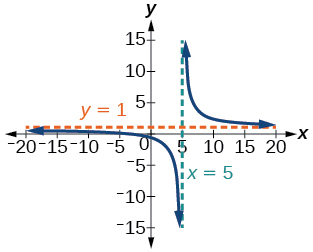$f\left(x\right)=\frac{{x}^{2}+1}{{x}^{2}-4}$

$f\left(x\right)=\frac{3{x}^{2}-27}{{x}^{2}+x-2}$

Intercepts (3, 0), (-3, 0), and $\text{\hspace{0.17em}}\left(0,\frac{27}{2}\right)\text{\hspace{0.17em}}$ , Asymptotes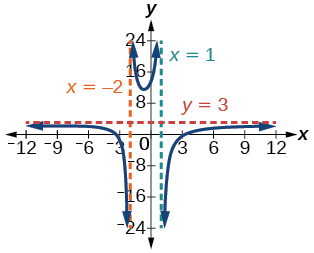$f\left(x\right)=\frac{x+2}{{x}^{2}-9}$

For the following exercises, find the slant asymptote.

$f\left(x\right)=\frac{{x}^{2}-1}{x+2}$

$f\left(x\right)=\frac{2{x}^{3}-{x}^{2}+4}{{x}^{2}+1}$

## Inverses and Radical Functions

For the following exercises, find the inverse of the function with the domain given.

$f\left(x\right)={\left(x-2\right)}^{2},\text{\hspace{0.17em}}x\ge 2$

${f}^{-1}\left(x\right)=\sqrt{x}+2$

$f\left(x\right)={\left(x+4\right)}^{2}-3,\text{\hspace{0.17em}}x\ge -4$

$f\left(x\right)={x}^{2}+6x-2,\text{\hspace{0.17em}}x\ge -3$

${f}^{-1}\left(x\right)=\sqrt{x+11}-3$

$f\left(x\right)=2{x}^{3}-3$

$f\left(x\right)=\sqrt{4x+5}-3$

${f}^{-1}\left(x\right)=\frac{{\left(x+3\right)}^{2}-5}{4},\text{\hspace{0.17em}}x\ge -3$

$f\left(x\right)=\frac{x-3}{2x+1}$

## Modeling Using Variation

For the following exercises, find the unknown value.

$\text{\hspace{0.17em}}y\text{\hspace{0.17em}}$ varies directly as the square of $\text{\hspace{0.17em}}x.\text{\hspace{0.17em}}$ If when find $\text{\hspace{0.17em}}y\text{\hspace{0.17em}}$ if $\text{\hspace{0.17em}}x=4.$

$y=64$

$\text{\hspace{0.17em}}y\text{\hspace{0.17em}}$ varies inversely as the square root of $\text{\hspace{0.17em}}x\text{\hspace{0.17em}}$ If when find $\text{\hspace{0.17em}}y\text{\hspace{0.17em}}$ if $\text{\hspace{0.17em}}x=4.$

$\text{\hspace{0.17em}}y\text{\hspace{0.17em}}$ varies jointly as the cube of $\text{\hspace{0.17em}}x\text{\hspace{0.17em}}$ and as $\text{\hspace{0.17em}}z.\text{\hspace{0.17em}}$ If when $\text{\hspace{0.17em}}x=1\text{\hspace{0.17em}}$ and $\text{\hspace{0.17em}}z=2,\text{\hspace{0.17em}}$ $y=6,\text{\hspace{0.17em}}$ find $\text{\hspace{0.17em}}y\text{\hspace{0.17em}}$ if $\text{\hspace{0.17em}}x=2\text{\hspace{0.17em}}$ and $\text{\hspace{0.17em}}z=3.$

$\text{\hspace{0.17em}}y\text{\hspace{0.17em}}$ varies jointly as $\text{\hspace{0.17em}}x\text{\hspace{0.17em}}$ and the square of $\text{\hspace{0.17em}}z\text{\hspace{0.17em}}$ and inversely as the cube of $\text{\hspace{0.17em}}w.\text{\hspace{0.17em}}$ If when $\text{\hspace{0.17em}}x=3,\text{\hspace{0.17em}}$ $z=4,\text{\hspace{0.17em}}$ and $\text{\hspace{0.17em}}w=2,\text{\hspace{0.17em}}$ $y=48,\text{\hspace{0.17em}}$ find $\text{\hspace{0.17em}}y\text{\hspace{0.17em}}$ if $\text{\hspace{0.17em}}x=4,\text{\hspace{0.17em}}$ $z=5,\text{\hspace{0.17em}}$ and $\text{\hspace{0.17em}}w=3.$

For the following exercises, solve the application problem.

The weight of an object above the surface of the earth varies inversely with the distance from the center of the earth. If a person weighs 150 pounds when he is on the surface of the earth (3,960 miles from center), find the weight of the person if he is 20 miles above the surface.

148.5 pounds

The volume $\text{\hspace{0.17em}}V\text{\hspace{0.17em}}$ of an ideal gas varies directly with the temperature $\text{\hspace{0.17em}}T\text{\hspace{0.17em}}$ and inversely with the pressure P. A cylinder contains oxygen at a temperature of 310 degrees K and a pressure of 18 atmospheres in a volume of 120 liters. Find the pressure if the volume is decreased to 100 liters and the temperature is increased to 320 degrees K.

## Chapter test

Give the degree and leading coefficient of the following polynomial function.

$f\left(x\right)={x}^{3}\left(3-6{x}^{2}-2{x}^{2}\right)$

Determine the end behavior of the polynomial function.

$f\left(x\right)=8{x}^{3}-3{x}^{2}+2x-4$

$As\text{\hspace{0.17em}}x\to -\infty ,\text{\hspace{0.17em}}f\left(x\right)\to -\infty ,\text{\hspace{0.17em}}as\text{\hspace{0.17em}}x\to \infty ,\text{\hspace{0.17em}}f\left(x\right)\to \infty$

$f\left(x\right)=-2{x}^{2}\left(4-3x-5{x}^{2}\right)$

Write the quadratic function in standard form. Determine the vertex and axes intercepts and graph the function.

$f\left(x\right)={x}^{2}+2x-8$

$f\left(x\right)={\left(x+1\right)}^{2}-9$ , vertex $\text{\hspace{0.17em}}\left(-1,-9\right)$ , intercepts $\text{\hspace{0.17em}}\left(2,0\right);\left(-4,0\right);\text{\hspace{0.17em}}\left(0,-8\right)$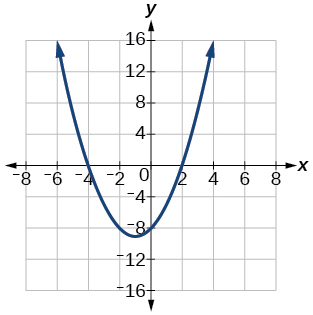Given information about the graph of a quadratic function, find its equation.

Vertex $\text{\hspace{0.17em}}\left(2,0\right)\text{\hspace{0.17em}}$ and point on graph $\text{\hspace{0.17em}}\left(4,12\right).$

Solve the following application problem.

A rectangular field is to be enclosed by fencing. In addition to the enclosing fence, another fence is to divide the field into two parts, running parallel to two sides. If 1,200 feet of fencing is available, find the maximum area that can be enclosed.

60,000 square feet

Find all zeros of the following polynomial functions, noting multiplicities.

$f\left(x\right)={\left(x-3\right)}^{3}\left(3x-1\right){\left(x-1\right)}^{2}$

$f\left(x\right)=2{x}^{6}-12{x}^{5}+18{x}^{4}$

0 with multiplicity 4, 3 with multiplicity 2

Based on the graph, determine the zeros of the function and multiplicities.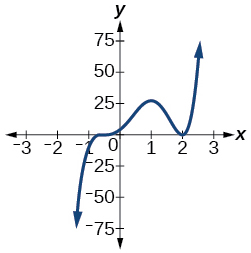Use long division to find the quotient.

$\frac{2{x}^{3}+3x-4}{x+2}$

$2{x}^{2}-4x+11-\frac{26}{x+2}$

Use synthetic division to find the quotient. If the divisor is a factor, write the factored form.

$\frac{{x}^{4}+3{x}^{2}-4}{x-2}$

$\frac{2{x}^{3}+5{x}^{2}-7x-12}{x+3}$

$2{x}^{2}-x-4$ . So factored form is $\text{\hspace{0.17em}}\left(x+3\right)\left(2{x}^{2}-x-4\right)$

Use the Rational Zero Theorem to help you find the zeros of the polynomial functions.

$f\left(x\right)=2{x}^{3}+5{x}^{2}-6x-9$

$f\left(x\right)=4{x}^{4}+8{x}^{3}+21{x}^{2}+17x+4$

$-\frac{1}{2}\text{\hspace{0.17em}}$ (has multiplicity 2), $\text{\hspace{0.17em}}\frac{-1±i\sqrt{15}}{2}\text{\hspace{0.17em}}$

$f\left(x\right)=4{x}^{4}+16{x}^{3}+13{x}^{2}-15x-18$

$f\left(x\right)={x}^{5}+6{x}^{4}+13{x}^{3}+14{x}^{2}+12x+8$

$\text{\hspace{0.17em}}-2\text{\hspace{0.17em}}$ (has multiplicity 3), $\text{\hspace{0.17em}}±i$

Given the following information about a polynomial function, find the function.

It has a double zero at $\text{\hspace{0.17em}}x=3\text{\hspace{0.17em}}$ and zeros at $\text{\hspace{0.17em}}x=1\text{\hspace{0.17em}}$ and $\text{\hspace{0.17em}}x=-2\text{\hspace{0.17em}}$ . Its y -intercept is $\text{\hspace{0.17em}}\left(0,12\right).\text{\hspace{0.17em}}$

It has a zero of multiplicity 3 at $\text{\hspace{0.17em}}x=\frac{1}{2}\text{\hspace{0.17em}}$ and another zero at $\text{\hspace{0.17em}}x=-3\text{\hspace{0.17em}}$ . It contains the point $\text{\hspace{0.17em}}\left(1,8\right).$

$f\left(x\right)=2{\left(2x-1\right)}^{3}\left(x+3\right)$

Use Descartes’ Rule of Signs to determine the possible number of positive and negative solutions.

$8{x}^{3}-21{x}^{2}+6=0$

For the following rational functions, find the intercepts and horizontal and vertical asymptotes, and sketch a graph.

$f\left(x\right)=\frac{x+4}{{x}^{2}-2x-3}$

Intercepts $\text{\hspace{0.17em}}\left(-4,0\right),\text{\hspace{0.17em}}\left(0,-\frac{4}{3}\right)\text{\hspace{0.17em}}$ , Asymptotes .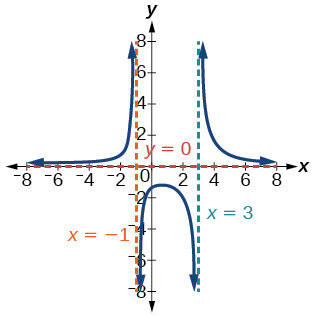$f\left(x\right)=\frac{{x}^{2}+2x-3}{{x}^{2}-4}$

Find the slant asymptote of the rational function.

$f\left(x\right)=\frac{{x}^{2}+3x-3}{x-1}$

$y=x+4$

Find the inverse of the function.

$f\left(x\right)=\sqrt{x-2}+4$

$f\left(x\right)=3{x}^{3}-4$

${f}^{-1}\left(x\right)=\sqrt{\frac{x+4}{3}}$

$f\left(x\right)=\frac{2x+3}{3x-1}$

Find the unknown value.

$\text{\hspace{0.17em}}y\text{\hspace{0.17em}}$ varies inversely as the square of $\text{\hspace{0.17em}}x\text{\hspace{0.17em}}$ and when $\text{\hspace{0.17em}}x=3,\text{\hspace{0.17em}}$ $y=2.\text{\hspace{0.17em}}$ Find $\text{\hspace{0.17em}}y\text{\hspace{0.17em}}$ if $\text{\hspace{0.17em}}x=1.$

$y=18$

$\text{\hspace{0.17em}}y\text{\hspace{0.17em}}$ varies jointly with $\text{\hspace{0.17em}}x\text{\hspace{0.17em}}$ and the cube root of $\text{\hspace{0.17em}}z.\text{\hspace{0.17em}}$ If when $\text{\hspace{0.17em}}x=2\text{\hspace{0.17em}}$ and $\text{\hspace{0.17em}}z=27,\text{\hspace{0.17em}}$ $y=12,\text{\hspace{0.17em}}$ find $\text{\hspace{0.17em}}y\text{\hspace{0.17em}}$ if $\text{\hspace{0.17em}}x=5\text{\hspace{0.17em}}$ and $\text{\hspace{0.17em}}z=8.$

Solve the following application problem.

The distance a body falls varies directly as the square of the time it falls. If an object falls 64 feet in 2 seconds, how long will it take to fall 256 feet?

4 seconds

#### Questions & Answers

A laser rangefinder is locked on a comet approaching Earth. The distance g(x), in kilometers, of the comet after x days, for x in the interval 0 to 30 days, is given by g(x)=250,000csc(π30x). Graph g(x) on the interval [0, 35]. Evaluate g(5)  and interpret the information. What is the minimum distance between the comet and Earth? When does this occur? To which constant in the equation does this correspond? Find and discuss the meaning of any vertical asymptotes.
Kaitlyn Reply
The sequence is {1,-1,1-1.....} has
amit Reply
circular region of radious
Kainat Reply
how can we solve this problem
Joel Reply
Sin(A+B) = sinBcosA+cosBsinA
Eseka Reply
Prove it
Eseka
Please prove it
Eseka
hi
Joel
June needs 45 gallons of punch. 2 different coolers. Bigger cooler is 5 times as large as smaller cooler. How many gallons in each cooler?
Arleathia Reply
7.5 and 37.5
Nando
find the sum of 28th term of the AP 3+10+17+---------
Prince Reply
I think you should say "28 terms" instead of "28th term"
Vedant
the 28th term is 175
Nando
192
Kenneth
if sequence sn is a such that sn>0 for all n and lim sn=0than prove that lim (s1 s2............ sn) ke hole power n =n
SANDESH Reply
write down the polynomial function with root 1/3,2,-3 with solution
Gift Reply
if A and B are subspaces of V prove that (A+B)/B=A/(A-B)
Pream Reply
write down the value of each of the following in surd form a)cos(-65°) b)sin(-180°)c)tan(225°)d)tan(135°)
Oroke Reply
Prove that (sinA/1-cosA - 1-cosA/sinA) (cosA/1-sinA - 1-sinA/cosA) = 4
kiruba Reply
what is the answer to dividing negative index
Morosi Reply
In a triangle ABC prove that. (b+c)cosA+(c+a)cosB+(a+b)cisC=a+b+c.
Shivam Reply
give me the waec 2019 questions
Aaron Reply

### Read also:

#### Get the best Algebra and trigonometry course in your pocket!

Source:  OpenStax, Algebra and trigonometry. OpenStax CNX. Nov 14, 2016 Download for free at https://legacy.cnx.org/content/col11758/1.6
Google Play and the Google Play logo are trademarks of Google Inc.

Notification Switch

Would you like to follow the 'Algebra and trigonometry' conversation and receive update notifications?ByByBy Lakeima RobertsBy Anonymous UserBy RhodesBy Abishek DevarajBy Edward Biton